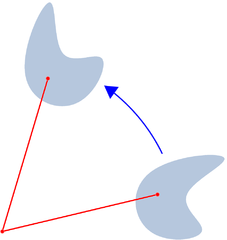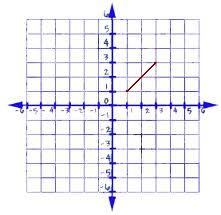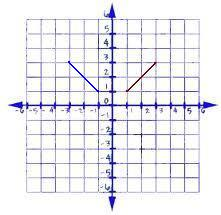# Rotations in Math: Definition & Overview

An error occurred trying to load this video.

Try refreshing the page, or contact customer support.

Coming up next: Similar Shapes in Math: Definition & Overview

### You're on a roll. Keep up the good work!

Replay
Your next lesson will play in 10 seconds
• 0:01 Geometric Transformations
• 0:39 Rotations
• 1:26 Notation
• 1:53 Rules of Rotation
• 3:55 Examples
• 4:39 Lesson Summary

Want to watch this again later?

Timeline
Autoplay
Autoplay
Speed

#### Recommended Lessons and Courses for You

Lesson Transcript
Instructor: Jennifer Beddoe
A geometric rotation refers to the rotating of a figure around a center of rotation. This lesson will get you going on rotations, give you some examples, and end with a quiz that tests your knowledge of rotations.

## Geometric Transformations

There are four types of geometric transformations:

1. Translation - movement of the object without rotating it or changing its size
2. Reflection - flipping the object about a line of reflection
3. Rotation - rotating a figure about a point
4. Dilation - changing the size of a figure without changing its essential shape

For this lesson, we'll focus specifically on rotation.

## RotationsA rotation is the movement of a geometric figure about a certain point. The amount of rotation is described in terms of degrees. If the degrees are positive, the rotation is performed counterclockwise; if they are negative, the rotation is clockwise. The figure will not change size or shape, but, unlike a translation, will change direction. The initial figure is always called the pre-image, while the rotated figure will be called the image.

Let's look at an example. Let's rotate this figure 90 degrees:When the pre-image is rotated, the final image looks like this, where the maroon-colored line is the pre-image and the blue line is the image:## Notation

The mathematical notation for rotation is usually written like this: R (center, rotation), where the center is the point of rotation and the rotation is given in degrees. Often, rotations are written using coordinate notation, which means that their coordinates on the coordinate plane are given. This will help you to draw both the pre-image and the image easily.

## Rules of Rotation

There are some general rules for the rotation of objects using the most common degree measures (90 degrees, 180 degrees, and 270 degrees). The general rule for rotation of an object 90 degrees is (x, y) --------> (-y, x). You can use this rule to rotate a pre-image by taking the points of each vertex, translating them according to the rule, and drawing the image. Take the previous example: the points that mark the ends of the pre-image are (1, 1) and (3, 3). When you rotate the image using the 90 degrees rule, the end points of the image will be (-1, 1) and (-3, 3).

The rules for the other common degree rotations are:

• For 180 degrees, the rule is (x, y) --------> (-x, -y)
• For 270 degrees, the rule is (x, y) --------> (y, -x)

To unlock this lesson you must be a Study.com Member.

### Register to view this lesson

Are you a student or a teacher?

### Unlock Your Education

#### See for yourself why 30 million people use Study.com

##### Become a Study.com member and start learning now.
Back
What teachers are saying about Study.com

### Earning College Credit

Did you know… We have over 160 college courses that prepare you to earn credit by exam that is accepted by over 1,500 colleges and universities. You can test out of the first two years of college and save thousands off your degree. Anyone can earn credit-by-exam regardless of age or education level.